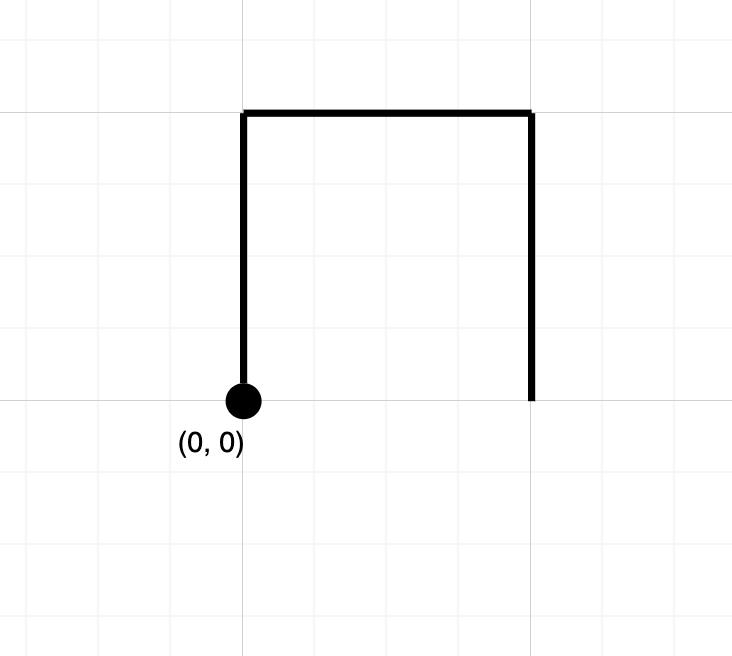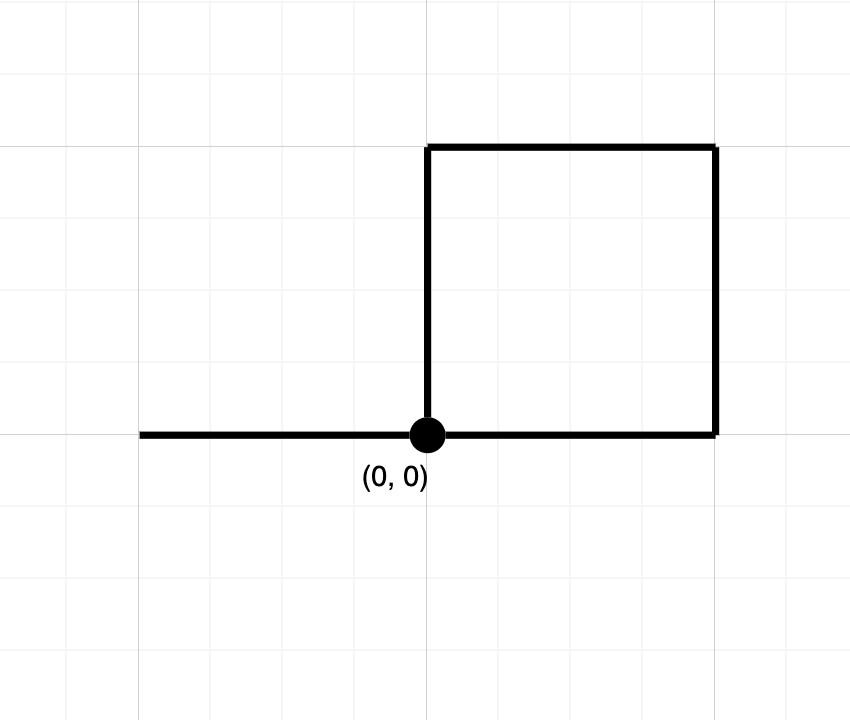# 1496. Path Crossing

Easy
Given a string `path`, where `path[i] = 'N'`, `'S'`, `'E'` or `'W'`, each representing moving one unit north, south, east, or west, respectively. You start at the origin `(0, 0)` on a 2D plane and walk on the path specified by `path`.
Return `true` if the path crosses itself at any point, that is, if at any time you are on a location you have previously visited. Return `false` otherwise.
Example 1:Input: path = "NES"
Output:
false
Explanation:
Notice that the path doesn't cross any point more than once.
Example 2:Input: path = "NESWW"
Output:
true
Explanation:
Notice that the path visits the origin twice.
Constraints:
• `1 <= path.length <= 104`
• `path[i]` is either `'N'`, `'S'`, `'E'`, or `'W'`.

### 解題

Runtime: 0 ms, faster than 100.00%
Memory Usage: 2.2 MB, less than 26.09%
func isPathCrossing(path string) bool {
visited := make(map[int]bool)
visited[int{ 0, 0 }] = true
x, y := 0, 0
for _, char := range path {
if char== 'N' {
y++
} else if char=='E' {
x++
} else if char=='S' {
y--
} else {
x--
}
if _, ok := visited[int{x, y}]; ok {
return true
} else {
visited[int{x, y}] = true
}
}
return false
}